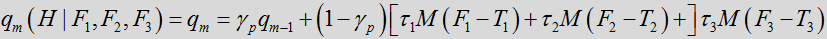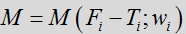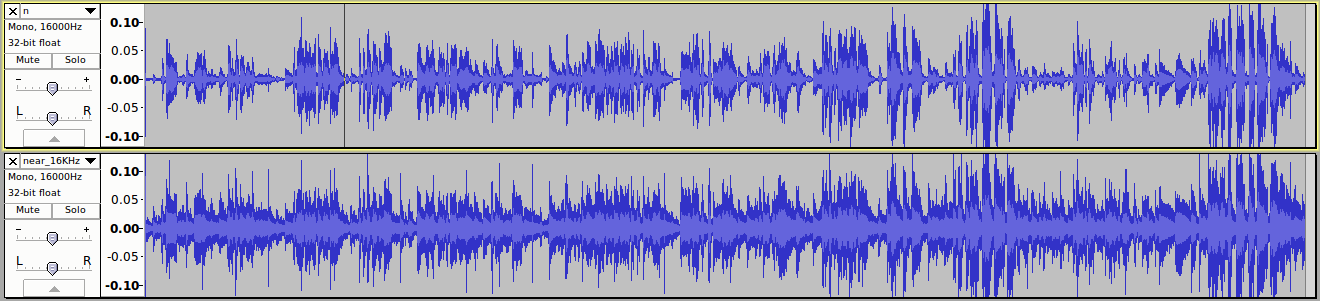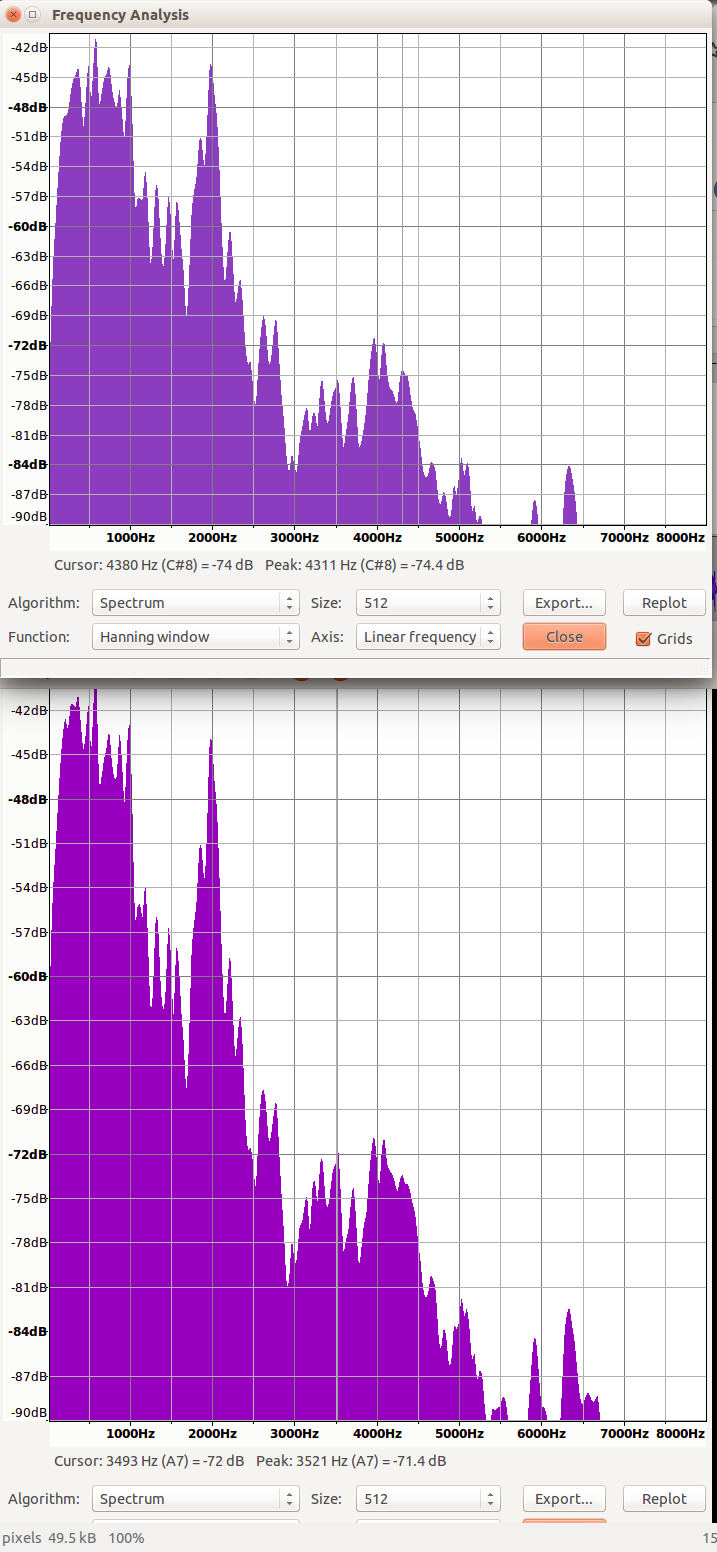2016-09-20 12:39:01 shichaog 阅读数 20112
• ###### Mikrotik RouterOS 软路由基础教程

基础课程，给大家对Mikrotik RouterOS 有一个基础的认识，并且对于新入门的网络管理人员，网络工程师来说，提供更快速更好的开箱即用的技术交付。 降低网络管理的工作强度，提升工作的效率。 通过学习，朋友们能自行横向对比思科，华为，还有一些国内的企业路由的，理解Mikrotik 路由器的实际优势。 本教程带了基础的原理讲解和实例操作，在我的博客里面都有对应的文章，欢迎大家CSND博客里面留言提问，一起成长。

409 人正在学习 去看看 梁灿

CSND已永久停更，最新版唯一来源点击下面链接跳转：

WebRTC噪声抑制核心算法在ns_core.c文件里。

## 算法原理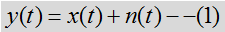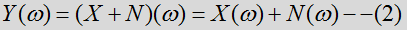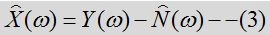2.基于全局幅度谱最小原理，该估计认为幅度谱最小的情况必然对应没有语音的时候。

3.还有基于矩阵奇异值分解原理估计噪声的

对接收到的每一帧带噪语音信号，以对该帧的初始噪声估计为前提，定义语音概率函数，测量每一帧带噪信号的分类特征，使用测量出来的分类特征，计算每一帧基于多特征的语音概率，在对计算出的语音概率进行动态因子（信号分类特征和阈值参数）加权，根据计算出的每帧基于特征的语音概率，修改多帧中每一帧的语音概率函数，以及使用修改后每帧语音概率函数，更新每帧中的初始噪声（连续多帧中每一帧的分位数噪声）估计。

## WebRtcNs_InitCore

NS（noise suppression）模块初始化，后面的代码按fs=8000，来分析。

``````//语音数据的长度，8k/10ms的数据量是80
self->blockLen = 80;
//分析长度，由于是在频域分析，将长度像上取2的幂次，最小的值是128，实际上是fft的长度。
self->anaLen = 128;
//窗函数，采用混合汉宁平顶窗函数
self->window = kBlocks80w128;``````

``````  // Initialize FFT work arrays.
self->ip = 0;  // Setting this triggers initialization.
memset(self->dataBuf, 0, sizeof(float) * ANAL_BLOCKL_MAX);
WebRtc_rdft(self->anaLen, 1, self->dataBuf, self->ip, self->wfft);

//是滑动分析窗，针对80点128的fft而言，每一次会保留前一帧的128-80=48个点的数据，而不是对80点简单填充0变成128点做fft。
//但这会带来合成上的问题，通常采用加窗以防止重叠带来的突变。可以使用做fft变换一样的窗函数。但这要求窗函数保幂映射，即重叠
//区部分窗口的平方和必须为1.
memset(self->analyzeBuf, 0, sizeof(float) * ANAL_BLOCKL_MAX);
//dataBuf存储的是原始时域信号
memset(self->dataBuf, 0, sizeof(float) * ANAL_BLOCKL_MAX);
//syntBuf是谱减法，减去噪声后变换到时域的信号
memset(self->syntBuf, 0, sizeof(float) * ANAL_BLOCKL_MAX);

// For HB processing. 这是高频部分，最多有两个band，
memset(self->dataBufHB,
0,
sizeof(float) * NUM_HIGH_BANDS_MAX * ANAL_BLOCKL_MAX);``````

``````  // For quantile noise estimation.
memset(self->quantile, 0, sizeof(float) * HALF_ANAL_BLOCKL);
//3帧同步估计，lquantile是对数分位数。density是概率密度，计算分位数用到概率密度的。
for (i = 0; i < SIMULT * HALF_ANAL_BLOCKL; i++) {
self->lquantile[i] = 8.f;
self->density[i] = 0.3f;
}

for (i = 0; i < SIMULT; i++) {
//我的理解counter是一个权值，代表的每一帧对分位数估计而言其所占的比重。
self->counter[i] =
(int)floor((float)(END_STARTUP_LONG * (i + 1)) / (float)SIMULT);
}

``````  for (i = 0; i < HALF_ANAL_BLOCKL; i++) {
self->smooth[i] = 1.f;
}``````

``````  // Set the aggressiveness: default.
self->aggrMode = 0;``````

``````  // Initialize variables for new method.
self->priorSpeechProb = 0.5f;  // Prior prob for speech/noise.
// Previous analyze mag spectrum.
memset(self->magnPrevAnalyze, 0, sizeof(float) * HALF_ANAL_BLOCKL);
// Previous process mag spectrum.
memset(self->magnPrevProcess, 0, sizeof(float) * HALF_ANAL_BLOCKL);
// Current noise-spectrum.
memset(self->noise, 0, sizeof(float) * HALF_ANAL_BLOCKL);
// Previous noise-spectrum.
memset(self->noisePrev, 0, sizeof(float) * HALF_ANAL_BLOCKL);
// Conservative noise spectrum estimate.
memset(self->magnAvgPause, 0, sizeof(float) * HALF_ANAL_BLOCKL);
// For estimation of HB in second pass.
memset(self->speechProb, 0, sizeof(float) * HALF_ANAL_BLOCKL);
// Initial average magnitude spectrum.
memset(self->initMagnEst, 0, sizeof(float) * HALF_ANAL_BLOCKL);
for (i = 0; i < HALF_ANAL_BLOCKL; i++) {
// Smooth LR (same as threshold).
self->logLrtTimeAvg[i] = LRT_FEATURE_THR;
}``````

``````  // Feature quantities.
// Spectral flatness (start on threshold).
self->featureData = SF_FEATURE_THR;
self->featureData = 0.f;  // Spectral entropy: not used in this version.
self->featureData = 0.f;  // Spectral variance: not used in this version.
// Average LRT factor (start on threshold).
self->featureData = LRT_FEATURE_THR;
// Spectral template diff (start on threshold).
self->featureData = SF_FEATURE_THR;
self->featureData = 0.f;  // Normalization for spectral difference.
// Window time-average of input magnitude spectrum.
self->featureData = 0.f;

// Histogram quantities: used to estimate/update thresholds for features.
memset(self->histLrt, 0, sizeof(int) * HIST_PAR_EST);
memset(self->histSpecFlat, 0, sizeof(int) * HIST_PAR_EST);
memset(self->histSpecDiff, 0, sizeof(int) * HIST_PAR_EST);

// Update flag for parameters:
// 0 no update, 1 = update once, 2 = update every window.
self->modelUpdatePars = 2;
self->modelUpdatePars = 500;  // Window for update.
// Counter for update of conservative noise spectrum.
self->modelUpdatePars = 0;
// Counter if the feature thresholds are updated during the sequence.
self->modelUpdatePars = self->modelUpdatePars;
``````

``````  self->signalEnergy = 0.0;
self->sumMagn = 0.0;
self->whiteNoiseLevel = 0.0;
self->pinkNoiseNumerator = 0.0;
self->pinkNoiseExp = 0.0;``````

## ComputeSpectralFlatness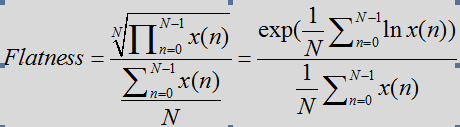``````// Compute spectral flatness on input spectrum.
// |magnIn| is the magnitude spectrum.
// Spectral flatness is returned in self->featureData.
static void ComputeSpectralFlatness(NoiseSuppressionC* self,
const float* magnIn) {
size_t i;
size_t shiftLP = 1;  // Option to remove first bin(s) from spectral measures.
float avgSpectralFlatnessNum, avgSpectralFlatnessDen, spectralTmp;

// Compute spectral measures.
// For flatness.
avgSpectralFlatnessNum = 0.0;
avgSpectralFlatnessDen = self->sumMagn;
for (i = 0; i < shiftLP; i++) {
//跳过第一个频点，即直流频点Den是denominator（分母）的缩写，avgSpectralFlatnessDen是上述公式分母计算用到的
avgSpectralFlatnessDen -= magnIn[i];
}
// Compute log of ratio of the geometric to arithmetic mean: check for log(0) case.
// 计算分子部分，numerator(分子)，对log(0)是无穷小的值，所以计算时对这一情况特殊处理。
for (i = shiftLP; i < self->magnLen; i++) {
if (magnIn[i] > 0.0) {
avgSpectralFlatnessNum += (float)log(magnIn[i]);
} else {
//TVAG是time-average的缩写，对于能量出现异常的处理。利用前一次平坦度直接取平均返回。这里平滑因子是0.3.
self->featureData -= SPECT_FL_TAVG * self->featureData;
return;
}
}
// Normalize.
avgSpectralFlatnessDen = avgSpectralFlatnessDen / self->magnLen;
avgSpectralFlatnessNum = avgSpectralFlatnessNum / self->magnLen;

// Ratio and inverse log: check for case of log(0).
spectralTmp = (float)exp(avgSpectralFlatnessNum) / avgSpectralFlatnessDen;

// Time-avg update of spectral flatness feature.
self->featureData += SPECT_FL_TAVG * (spectralTmp - self->featureData);
// Done with flatness feature.
}
``````

## ComputeSpectralDifference

``  // avgDiffNormMagn = var(magnIn) - cov(magnIn, magnAvgPause)^2 / var(magnAvgPause)``

## ComputeSnr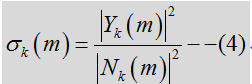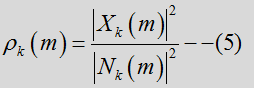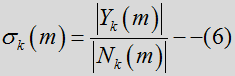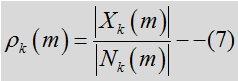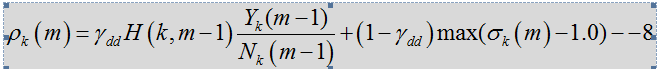``````static void ComputeSnr(const NoiseSuppressionC* self,
const float* magn,
const float* noise,
float* snrLocPrior,
float* snrLocPost) {
size_t i;

for (i = 0; i < self->magnLen; i++) {
// Previous post SNR.
// Previous estimate: based on previous frame with gain filter. 此处公式7加了平滑，对应公式8的前半部分
float previousEstimateStsa = self->magnPrevAnalyze[i] /
(self->noisePrev[i] + 0.0001f) * self->smooth[i];
// Post SNR.
snrLocPost[i] = 0.f;
if (magn[i] > noise[i]) {
snrLocPost[i] = magn[i] / (noise[i] + 0.0001f) - 1.f;//实际上magn中包括语音和noise，减一是将噪声减去，获得后验snr
}
// DD estimate is sum of two terms: current estimate and previous estimate.
// Directed decision update of snrPrior.
snrLocPrior[i] =
DD_PR_SNR * previousEstimateStsa + (1.f - DD_PR_SNR) * snrLocPost[i];//此处是公式8的计算
}  // End of loop over frequencies.
}
``````

## SpeechNoiseProb

``````该函数参数的意义。
// |magn| is the input magnitude spectrum.输入信号幅度谱，包括信号和噪声
// |noise| is the noise spectrum.

// |snrLocPrior| is the prior SNR for each frequency.
// |snrLocPost| is the post SNR for each frequency.``````

``````  // Compute feature based on average LR factor.
// This is the average over all frequencies of the smooth log LRT.
logLrtTimeAvgKsum = 0.0;
for (i = 0; i < self->magnLen; i++) {
tmpFloat1 = 1.f + 2.f * snrLocPrior[i];
tmpFloat2 = 2.f * snrLocPrior[i] / (tmpFloat1 + 0.0001f);
besselTmp = (snrLocPost[i] + 1.f) * tmpFloat2;
self->logLrtTimeAvg[i] +=
LRT_TAVG * (besselTmp - (float)log(tmpFloat1) - self->logLrtTimeAvg[i]);
logLrtTimeAvgKsum += self->logLrtTimeAvg[i];
}
logLrtTimeAvgKsum = (float)logLrtTimeAvgKsum / (self->magnLen);
self->featureData = logLrtTimeAvgKsum;``````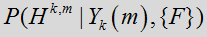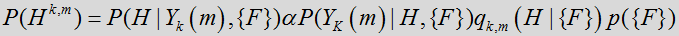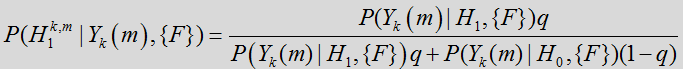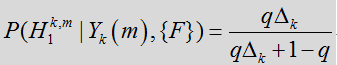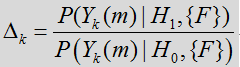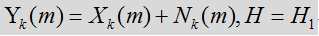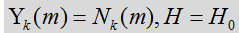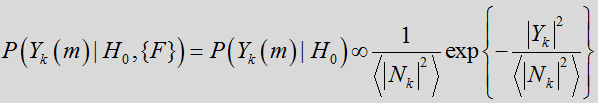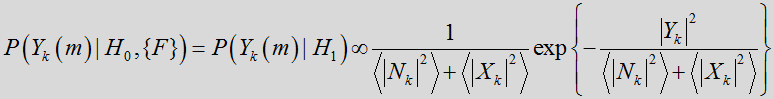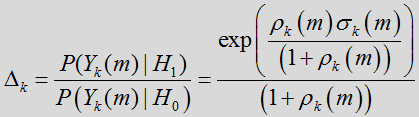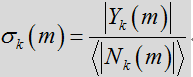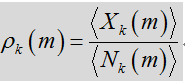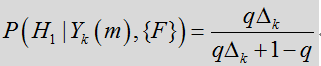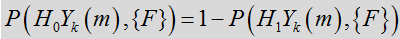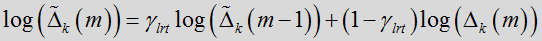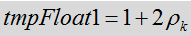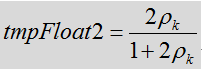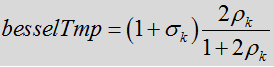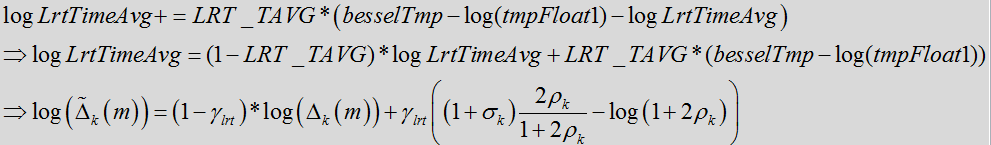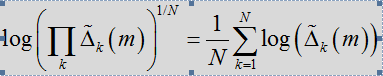`` logLrtTimeAvgKsum = (float)logLrtTimeAvgKsum / (self->magnLen);``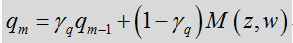1.LRT均值特征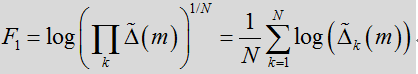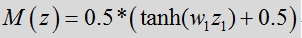``````  // Compute indicator function: sigmoid map.
indicator0 =
0.5f *
((float)tanh(widthPrior * (logLrtTimeAvgKsum - threshPrior0)) + 1.f);``````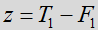2.频谱平坦度特征

为获得频谱平坦度特征，假设语音比噪声有更多的谐波行为。然而，语音频谱往往会在基频（基音）和谐波中出现峰值，而噪声频谱则相对平坦。因此，至少在某些布置中，本地频谱平坦度测量的综合可用做区分语音和噪声的良好判断依据。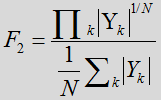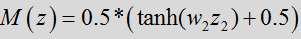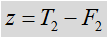``````  // Compute indicator function: sigmoid map.
indicator1 =
0.5f *
((float)tanh((float)sgnMap * widthPrior * (threshPrior1 - tmpFloat1)) +
1.f);``````

3.频谱模板差异特征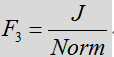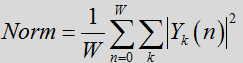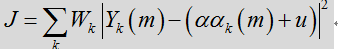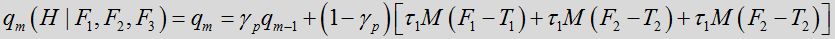``````  // Combine the indicator function with the feature weights.
indPrior = weightIndPrior0 * indicator0 + weightIndPrior1 * indicator1 +
weightIndPrior2 * indicator2;``````

``````  // Final speech probability: combine prior model with LR factor:.
gainPrior = (1.f - self->priorSpeechProb) / (self->priorSpeechProb + 0.0001f);
for (i = 0; i < self->magnLen; i++) {
invLrt = (float)exp(-self->logLrtTimeAvg[i]);
invLrt = (float)gainPrior * invLrt;
probSpeechFinal[i] = 1.f / (1.f + invLrt);
}``````

## 噪声估计 UpdateNoiseEstimate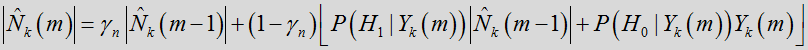``````    probSpeech = self->speechProb[i];
probNonSpeech = 1.f - probSpeech;
// Temporary noise update:
// Use it for speech frames if update value is less than previous.
noiseUpdateTmp = gammaNoiseTmp * self->noisePrev[i] +
(1.f - gammaNoiseTmp) * (probNonSpeech * magn[i] +
probSpeech * self->noisePrev[i]);``````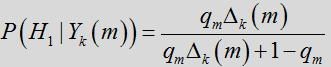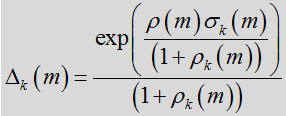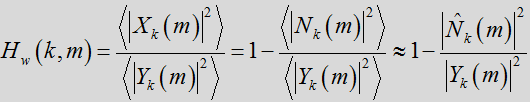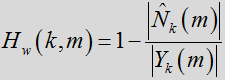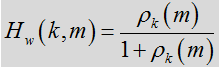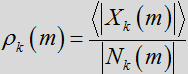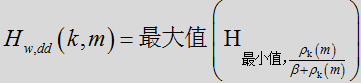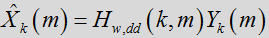## 信号合成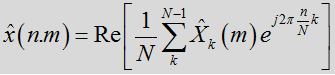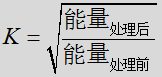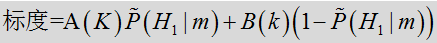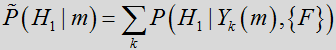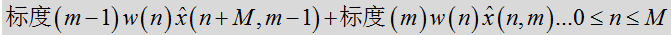## 参数估计